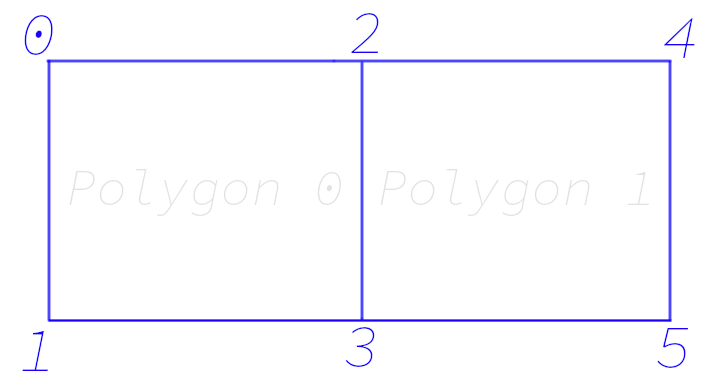Skip to content
{{ message }}

Instantly share code, notes, and snippets.

# AndreaCatania/FBX_edges.md

Last active Jan 14, 2021
Explanation on how the edges are stored into the FBX.

# FBX format - Unique Edges

#### How to parse the edges from FBX?

Let's consider this geometry:

``````Objects:  {
Geometry: 140696156120336, "Geometry::", "Mesh" {
Vertices: *18 {
a: -1,-1,0,1,-1,0,-1,1,0,1,1,0,-1.00631749629974,2.97582387924194,-0.0179548189043999,0.993682563304901,2.97582387924194,-0.0179548189043999
}
PolygonVertexIndex: *8 {
a: 0,1,3,-3,2,3,5,-5
}
Edges: *7 {
a: 0,1,2,3,5,6,7
}
``````The edges (stored as an array of 7 integers) is a direct reference to the `PolygonVertexIndex`. You can take the vertex of an edge in this way:

`int vertex_index = PolygonVertexIndex[Edges];`

The Edge array contains just the first vertex of each unique edge, the other vertex is the following vertex in the polygon, if the vertex is negative the first polygon vertex is its next. This mean that in our case we have the following edges:

```// Polygon 1
int polygon0_edge_0 = {PolygonVertexIndex[Edges], PolygonVertexIndex[Edges+1]}; // Vertex 0 - 1
int polygon0_edge_1 = {PolygonVertexIndex[Edges], PolygonVertexIndex[Edges+1]}; // Vertex 1 - 3
int polygon0_edge_2 = {PolygonVertexIndex[Edges], ~PolygonVertexIndex[Edges+1]}; // Vertex 3 - 2
int polygon0_edge_3 = {~PolygonVertexIndex[Edges], PolygonVertexIndex}; // Vertex 2 - 0

// Polygon 2
int polygon1_edge_0 = {PolygonVertexIndex[Edges], PolygonVertexIndex[Edges+1]}; // Vertex 3 - 5
int polygon1_edge_1 = {PolygonVertexIndex[Edges], ~PolygonVertexIndex[Edges+1]}; // Vertex 5 - 4
int polygon1_edge_2 = {~PolygonVertexIndex[Edges], PolygonVertexIndex}; // Vertex 4 - 2```

Note: You may have noticed the use of `~` in the code. It's used to reverse the integer and obtain the actual vertex ID.

to join this conversation on GitHub. Already have an account? Sign in to comment